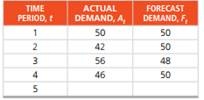Create an Account

Home / Questions / Consider the following actual (A t ) and forecast (F t ) demand levels for a commercial mu...

Consider the following actual (A t ) and forecast (F t ) demand levels for a commercial multiline...

Consider the following actual (At) and forecast (Ft) demand levels for a commercial multiline telephone at Office Max:The first forecast, F1, was derived by observing A1 and setting F1 equal to A1. Subsequent forecast averages were derived by exponential smoothing. Using the exponential smoothing method, find the forecast for time period 5. (Hint: You need to first find the smoothing constant, a.)

Jul 31 2020 View more View LessSubscribe To Get Solution